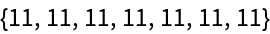#Function Repository Resource:

# PartitionRank

The largest part minus the number of parts of a partition

Contributed by: George Beck
 ResourceFunction["PartitionRank"][x] gives the difference of the largest part of the partition x and the number of parts of x.

## Details and Options

A partition of a positive integer n is a weakly decreasing list of positive integers that add up to to n.
The rank is a partition statistic useful in studying congruence properties of the partition function p(n), given by PartitionsP in the Wolfram Language.

## Examples

### Basic Examples

The largest part is 4 and there are 3 parts; 4-3=1:

 In:=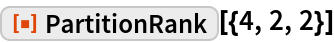Out=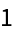The largest part and the number of parts are both 5; 5-5=0:

 In:=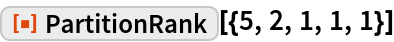Out=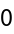### Neat Examples

Ramanujan discovered and proved analytically that the number of partitions of 5m+4 is divisible by 5:

 In:=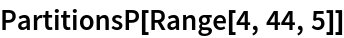Out=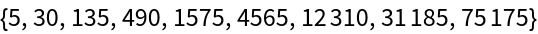Dyson conjectured and Atkins–Swinnerton–Dyer proved that the partitions of a number of the form 5m+4 can be split into five sets of equal size according to the rank mod 5:

 In:=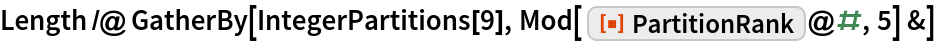Out=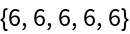Here is another example:

 In:=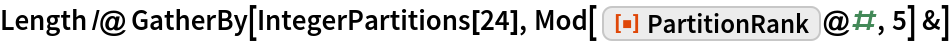Out=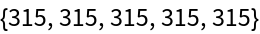A similar result holds for 7; in this case the partitions of a number of the form 7m+5 can be split into 7 sets of equal size, according to the rank mod 7:

 In:=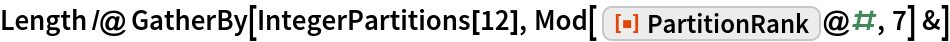Out=